# Joint Inference in Weakly-Annotated Image Datasets via Dense Correspondence

## Abstract

We present a principled framework for inferring pixel labels in weakly-annotated image datasets. Most previous, example-based approaches to computer vision rely on a large corpus of densely labeled images. However, for large, modern image datasets, such labels are expensive to obtain and are often unavailable. We establish a large-scale graphical model spanning all labeled and unlabeled images, then solve it to infer pixel labels jointly for all images in the dataset while enforcing consistent annotations over similar visual patterns. This model requires significantly less labeled data and assists in resolving ambiguities by propagating inferred annotations from images with stronger local visual evidences to images with weaker local evidences. We apply our proposed framework to two computer vision problems, namely image annotation with semantic segmentation, and object discovery and co-segmentation (segmenting multiple images containing a common object). Extensive numerical evaluations and comparisons show that our method consistently outperforms the state-of-the-art in automatic annotation and semantic labeling, while requiring significantly less labeled data. In contrast to previous co-segmentation techniques, our method manages to discover and segment objects well even in the presence of substantial amounts of noise images (images not containing the common object), as typical for datasets collected from Internet search.

## Introduction

Natural images consist of many repetitive patterns, such as corners, boundaries and textures, as well as repetitive parts, objects and scenes. Such repetitions occur not only within an image, but also across images. For example, when querying an image search engine using a textual phrase, we often obtain many visually similar images consisting of the object or scene of interest.

There are two main approaches in computer vision to model such visual repetitions. One approach—the parametric approach—explicitly learns a dictionary of visual patterns and their variations. Such parametric models have been successfully used for texture synthesis (Zhu et al. 1998), image denoising (Zoran and Weiss 2012), and object recognition (Fergus et al. 2003; Felzenszwalb et al. 2008). The other approach is the nonparametric approach, which attempts to build a graph for the patterns such that each pattern is connected to its lookalikes, also known as its “neighbors”. Information can then be conveniently propagated or transferred from the nearest neighbors to the query pattern without the need to explicitly model the pattern. Such methods have been widely used for super resolution (Freeman et al. 2000), texture synthesis (Liang et al. 2001), and image understanding (Liu et al. 2011a; Karsch et al. 2012), and, despite their relative simplicity, they often turn out to perform better their parametric, model-based counterparts.

Recent techniques for establishing dense correspondences between images of different scenes, such as SIFT flow (Liu et al. 2011b) and PatchMatch (Barnes et al. 2009), have facilitated the design and implementation of such nonparametric, information-transfer systems, where the information can be labels (Liu et al. 2011a), motion (Liu et al. 2011b), depth (Karsch et al. 2012), or pixel color (Tappen and Liu 2012). The general design of these systems is illustrated at the top of Fig. 1. For a query image x, the system first finds a set of images $$x_{i}$$ that are visually similar to x within a dataset of images, where each $$x_{i}$$ is associated with some known information $$y_{i}$$ (e.g. semantic labels, depth, or motion). After dense correspondence is established between x and each $$x_{i}$$, each $$y_{i}$$ is warped to x based on the computed correspondence, and an estimate of y for x is typically obtained by integrating multiple warped $$y_{i}$$’s. Such a system performs well in generating the function $$x\rightarrow y$$.

The main drawback of information-transfer methods, however, is that they rely on regularities in a large corpus of training images for which the information to be transferred (e.g. depth, motion, 3D) is “clean” and known. In large, modern image datasets, such information is expensive to obtain and is often noisy or unavailable. Moreover, when classifying multiple new images, these methods typically solve for each new image independently, which often results in inconsistent annotations across images due to visual ambiguities.

We therefore propose a new framework for dealing with weakly-annotated datasets. In such datasets, it may be that none of the closest neighbors of an image is labeled, and so traditional, correspondence-based approaches cannot be used. Instead, we gradually infer the pixel labels and propagate the information through the dataset jointly in all the images. In essence, our framework can be seen as an extension of the seminal work of Freeman et al. (2000) on learning models for low-level vision, to cases with scarce training data.

By means of dense image correspondence, we establish a large-scale Markov Random Field model (MRF) spanning all labeled and unlabeled images (Fig. 3), and infer pixel labels jointly for the entire dataset rather than for a single image. Pixel correspondences are used to capture the visual variability of semantically-related features across the dataset, and for inferring labels that are consistent over similar image patterns across different images.

Our model is effectively optimized by efficient belief propagation algorithms embedded within an expectation–maximization (EM) scheme, alternating between estimating the likelihood of pixel labels and pixel-wise label inference, while optionally refining the image graph structure during the optimization. Our optimization technique is not fundamentally new, but is designed to make full use of the available (sparse) annotations, and leverages modern computer architectures to scale efficiently for parallel computation.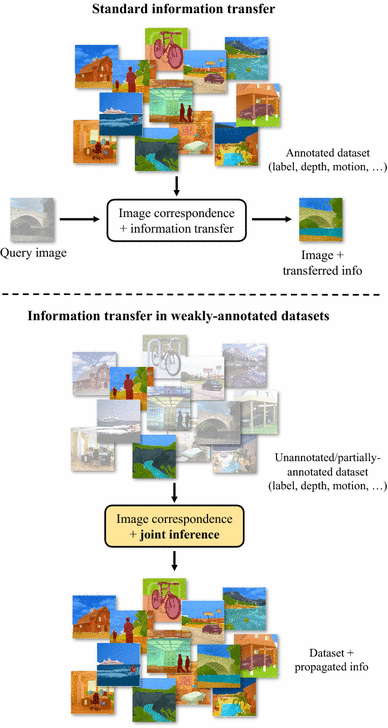Fig. 1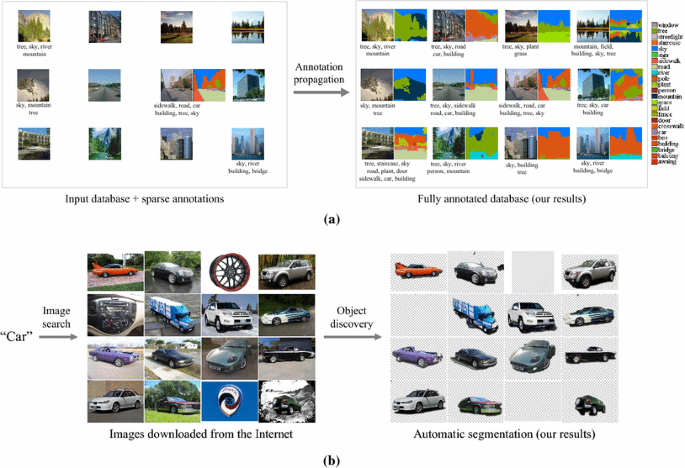Fig. 2

To illustrate the potential breadth of our framework, we apply it to two important computer vision applications (Fig. 2). The first is image annotation and sematic labeling, where the goal is to automatically annotate many images with a set of word tags and a pixel-wise map showing where each word tag occurs (Fig. 2a). The second is object discovery and segmentation, in which we seek to automatically segment multiple images containing a common object (Fig. 2b). We consider the first application in a weakly-labeled setup, where only sparse image-level tags and relatively few (or none) pixel labels are given. In the latter application, we assume that other than the fact that the image set contains some common object, there is no additional information available on the images or the common object class. Both applications can be useful for automatic generation of large-scale training sets for object detectors/classifiers, data-driven image synthesis, as well as for improving image-to-text relevance and Internet image search. They can also support applications in other domains, such as robotics, surveillance, and public safety.

We show how each of these two problems can be casted as a specific configuration of our proposed joint inference framework, and demonstrate that existing approaches to these problems do not perform well in more challenging, weakly-labeled scenarios. For image annotation, we conducted extensive experiments on standard large-scale datasets, namely LabelMe (Russell et al. 2008), ESP (Von Ahn and Dabbish 2004) and IAPR (Grubinger et al. 2006), showing that our system consistently outperforms the state-of-the-art in automatic annotation and semantic labeling, while requiring significantly less labeled data. For object discovery, our algorithm produces state-of-the-art results on the established MSRC and iCoseg co-segmentation datasets, and provides considerable improvement over previous co-segmentation methods on several new challenging Internet datasets containing rigid and non-rigid object categories.

The rest of the chapter is organized as follows. In Sect. 2 we review previous work related to our approach and the applications we explored. In Sect. 3 we formulate our framework. In Sects. 4 and 5 we apply the framework to the aforementioned applications. In each of these sections we first formulate the problem as a specific configuration of our framework, and then present experiments and results. We conclude our findings in Sect. 6.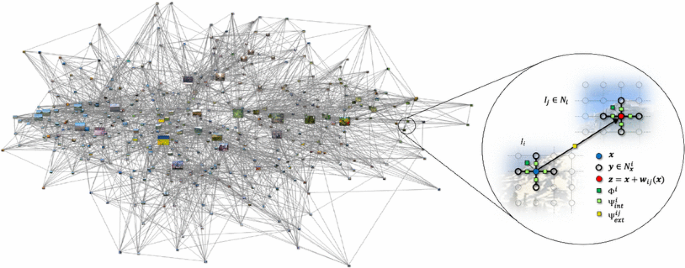Fig. 3

A prior version of this work appeared in the 12th European Conference on Computer Vision (ECCV), Florence 2012 (Rubinstein et al. 2012) and in the 26th IEEE Conference on Computer Vision and Pattern Recognition (CVPR), Portland 2013 (Rubinstein et al. 2013). Supplementary materials, additional results and code are available on the projet web pages: http://people.csail.mit.edu/mrub/annotation and http://people.csail.mit.edu/mrub/ObjectDiscovery.

## Related Work

Image Graphs Large-scale image graphs are becoming a fundamentally important representation for image datasets. Several works have utilized it in the past for exploring and navigating image collections. For example, “Image Webs” (Heath et al. 2010) discovers corresponding regions between images and uses spectral graph theory to capture the connectivity in an image dataset. The discovered connectivity is then used for revealing global structures in the dataset (such as paths linking between images), and for supporting a Photo-Tourism-style navigation (Snavely et al. 2006). Videoscapes (Tompkin et al. 2012) added the temporal domain to the image graph construction, aligning video frames not only in space, but also in time, in order to interactively explore unstructured video collections.

Recently, Liu et al. (2012) proposed a label propagation algorithm for joint image parsing, combining relationships among patches and mutual information in subgraphs of the image graph. Faktor and Irani (2012) efficiently estimate corresponding regions between images to automatically cluster an image dataset. Kim and Xing (2013) jointly discover matching images and parse their content in multiple photo streams to detect collective storylines. All these work, which were applied to specific problems, demonstrate the power of exploiting regularities and structures in image datasets to perform nontrivial inference tasks.

Image Annotation and Semantic Segmentation In computer vision, scholars have investigated image annotation in two directions. One methodology uses image similarities to transfer textual annotation from labeled images to unlabeled samples, under the assumption that similar images should have similar annotations. Notably, Makadia et al. (2010) recently proposed a simple baseline approach for auto-annotation based on global image features and a greedy algorithm for transferring tags from similar images. ARISTA (Wang et al. 2010) automatically annotates a web dataset of billions of images by transferring tags via near-duplicates. Although those methods have clearly demonstrated the merits of using similar images to transfer annotations, they do so only between globally very similar images, and cannot account for locally similar patterns.

Consequently, the other methodology focused on dense annotation of images, known as semantic labeling (Shotton et al. 2006, 2008; Liu et al. 2011a; Tighe and Lazebnik 2010), where correspondences between text and local image features are established for annotation propagation: similar local features should have similar labels. These methods often aim to label each pixel in an image using models learned from a training database. In Blei et al. (2003) and Feng et al. (2004), relationships between text and visual words are characterized by conventional language translation models. More recently, Shotton et al. (2006) proposed to train a discriminative model based on the texton representation, and to use conditional random fields (CRF) to combine various cues to generate spatially smooth labeling. The authors later extended their approach (Shotton et al. 2008) by using randomized decision forests for a significant speedup. Liu et al. (2011a) proposed a nonparametric approach to semantic labeling, where text labels are transferred from a labeled database to parse a query image via dense scene correspondences.

Since predicting annotation from image features is by nature ambiguous (e.g. textureless regions can be sky, wall, or ceiling), such methods rely on regularities in a large corpus of training data of pixel-wise densely labeled images. However, for large image databases, high-quality pixel labels are very expensive to obtain. Furthermore, the annotation is typically computed independently for each test image, which often results in inconsistent annotations due to visual ambiguities.

Object Discovery and Segmentation The task of simultaneously segmenting multiple images is known as Co-segmentation, where joint segmentation essentially serves as a means of compensating for the lack of supervisory data, allowing to infer the visual properties of the foreground object even in the absence of a priori information about the object or the images.

While numerous co-segmentation methods have been proposed, they were shown to work well mostly on small datasets, namely MSRC and iCoseg, containing salient and similar objects. In fact, in most of the images in those datasets the foreground can be quite easily separated from the background based on each image alone (i.e. without co-segmentation, see Sect. 5.4).

However, Internet image collections, such as the ones returned by image search engines for a given user query, are significantly larger and more diverse (Fig. 2b). Not only do the objects in images downloaded from the Internet exhibit drastically different style, color, texture, shape, pose, size, location and view-point; but such image collections also contain many noise images—images which do not contain the object of interest at all. These challenges, as we demonstrate, pose great difficulties on existing co-segmentation techniques. In particular, most co-segmentation methods assume every image contains the object of interest, and hence are unable to handle dataset noise.

Object discovery has been intensively studied in computer vision. In a supervised setup, objects were treated as topics and images as documents, and generative models such as Latent Dirichlet Allocation (LDA) and Hierarchical Pitman-Yor (HPY) have been used to learn the distribution and segmentation of multiple classes simultaneously (Sivic et al. 2005; Russell et al. 2006). Winn and Jojic (2005) propose a generative model for the distribution of mask, edge and color for visual objects with respect to a smooth deformation field. Although good object recovery results were reported, the model is limited to particular views of an object.

Recently, PageRank (Jing and Baluja 2008) was used to discover regions of interest in a bounding box representation (Kim and Torralba 2009), and self-similarities were used to discover a common pattern in several images (Bagon et al. 2010). Although in these works no generative models were used to learn the distribution of visual objects, reliable matching and saliency are found to be helpful for object discovery. The notions of matching and saliency were also successfully applied by Faktor and Irani (2012), a work done in parallel to ours, for unsupervised discovery of image categories.

Co-segmentation was first introduced by Rother et al. (2006), who used histogram matching to simultaneously segment the same object in two different images. Since then, numerous methods were proposed to improve and refine the co-segmentation (Mukherjee et al. 2009; Hochbaum and Singh 2009; Batra et al. 2010; Joulin et al. 2010), many of which work in the context of a pair of images with the exact same object (Rother et al. 2006; Mukherjee et al. 2009; Hochbaum and Singh 2009) or require some form of user interaction (Batra et al. 2010; Collins et al. 2012).

These techniques were later extended in various ways. Joulin et al. (2010) used a discriminative clustering framework that can handle multiple images, and Kim et al. (2011) proposed an optimization which scales up to even larger datasets. Vicente et al. (2011) introduced the notion of ”objectness” to the co-segmentation framework, showing that requiring the foreground segment to be an object often improves co-segmentation results significantly. All these techniques, however, maintain the strong assumption that the object is present in all of the images, which is not true for Internet image collections.

Other methods were proposed to handle images which might not contain the common object, either implicitly (Joulin et al. 2012) or explicitly (Kim and Xing 2012). In particular, Kim and Xing (2012) show promising results given additional user input, but do not show significant improvement in the unsupervised setting. It is clear that in the context of image search and web browsing, user input cannot be used.

Co-segmentation was also explored in weakly-supervised setups with multiple object categories (Rubinstein et al. 2012; Kuettel et al. 2012). While image annotations may facilitate object discovery and segmentation, image tags are often noisy, and bounding boxes or class labels are usually unavailable. In this work we show that it is plausible to automatically discover visual objects from the Internet using image search alone.

## Joint Inference via Dense Correspondence

In this section we describe our basic joint inference framework. We will start by defining the terminology and setup that will guide us through the rest of the chapter.

The input to our framework is a dataset $$\varvec{\Omega }=(\mathbf {I},\mathbf {V},\mathbf {A})$$ that is comprised of N RGB images $${\mathbf {I}}=\{I_1,\ldots ,I_N\}$$, a finite vocabulary $${\mathbf {V}} = \{l_1,\ldots ,l_L\}$$ of L possible labels each pixel can attain, and possibly additional image annotations $$\mathbf {A}=\{\mathbf {A}_1,\ldots ,\mathbf {A}_N\}$$. Image annotations can include a range of auxiliary information about an image. For example, it can be a collection of textual words describing the image, a time stamp specifying when the image was taken, GPS coordinates indicating where it was taken, and even pixel-level information, such as the location of faces or other objects in the image. In this chapter, we will consistently refer to semantic, pixel-level information as “labeling” and to textual, image-level annotation as “tags”. Our goal is to produce the labelings $${\mathbf {C}}=\{\mathbf {c}_1,\ldots ,\mathbf {c}_N\}$$ for all the images in the dataset, where for pixel $$\mathbf {x}=(x,y), \mathbf {c}_i({\mathbf {x}})\in \{1,\ldots ,L\}$$ indexes into the vocabulary $${\mathbf {V}}$$. We formulate this discrete labeling problem within an optimization framework to solve for the most likely labels for all pixels in the dataset.

To exploit the dataset structure and similarity between image regions, we establish correspondences between pixels in different images. We denote by $$\mathbf {w}_{ij}$$ the correspondence field—or flow field—from image $$I_i$$ to image $$I_j$$, mapping each pixel in $$I_i$$ to a pixel in $$I_j$$. For small datasets, we can estimate the correspondences between any pair of images, however for large datasets such computation is generally prohibitive. Therefore, we restrict the correspondences of each image $$I_i$$ to a subset of the images, $$\mathcal {N}_i$$, that are most similar to it, based on global image statistics that are more efficient to compute. In our experiments we fixed the size of $$\mathcal {N}_i$$ of each image $$I_i$$ to be the same constant, K, however in general this size can be allowed to vary. Finally, we denote by $$\mathbf {W}$$ the set of all pixel correspondences in the dataset: $$\mathbf {W}= \cup _{i=1}^N \cup _{I_j \in \mathcal {N}_i} \mathbf {w}_{ij}$$.

Given the input image dataset, $${\varvec{\varvec{\Omega }}}$$, the pixel correspondences $$\mathbf {W}$$, and additional parameters of the model, $$\varvec{\Theta }$$ (will be defined shortly), we define the cost function, $$E(\mathbf {C};{\varvec{\varvec{\Omega }}},\mathbf {W},\varvec{\Theta })$$ for the joint labeling $$\mathbf {C}$$, as:

\begin{aligned}&E({\mathbf {C}};{\varvec{\varvec{\Omega }}},{\mathbf {W}},{\varvec{\Theta }})\nonumber \\&\quad = \sum _{i=1}^N \sum _{\mathbf {x}\in \varLambda _i} \Big [\underbrace{\varPhi ^i(\mathbf {x})}_{\text {Likelihood (local evidence)}} + \underbrace{\varPhi ^i_{\theta }(\mathbf {x},\varvec{\Theta })}_{\text {Model parameters}} \nonumber \\&\quad + \underbrace{\sum _{\mathbf {y}\in \mathcal {N}^i_\mathbf {x}} \lambda _\textit{int}\varPsi _\textit{int}^i(\mathbf {x},\mathbf {y})}_{\text {Intra-image compatibility}} + \underbrace{\sum _{j \in \mathcal {N}_i} \lambda _\textit{ext}\varPsi _\textit{ext}^{ij}(\mathbf {x}, \mathbf {x}+ \mathbf {w}_{ij}(\mathbf {x}))}_{\text {Inter-image compatibility}} \Big ],\nonumber \\ \end{aligned}
(1)

where $$\mathcal {N}^i_\mathbf {x}$$ is the spatial neighbors of pixel $$\mathbf {x}$$ (we use the four pixels directly connect to $$\mathbf {x}$$ as the spatial neighborhood), and $$\varLambda _i$$ is image $$I_i$$’s lattice.

This objective function defines a (directed) graphical model over the entire image dataset (Fig. 3). The likelihood term, $$\varPhi ^i(x)$$, captures the cost of assigning the label $$\mathbf {c}_i(\mathbf {x})$$ to pixel $$\mathbf {x}$$ in image $$I_i$$. The definition of this term is problem-specific. $$\varPhi _\theta ^i$$ is a unary energy term that accounts for additional parameters of the model. In our implementations (will be described in the upcoming sections) these parameters include per-image color models, as well as dataset-wide parameters such as spatial distribution of labels, and label co-occurrences. These parameters are estimated during the optimization, and provide a simple mechanism to account for higher-order and longer-range connections between nodes in the graph (Freeman et al. 2000; Krähenbühl and Koltun 2012). The regularization terms, $$\varPsi ^i_{\textit{int}} \hbox { and } \varPsi ^{ij}_{\textit{ext}}$$, penalize discontinuous labeling within the image and between images, respectively, subject to image structures and similarities between corresponding pixels. $$\lambda _{\textit{int}} \hbox { and } \lambda _{\textit{ext}}$$ balance the contribution of these terms.

This graphical model extends traditional discrete MRF formulations used extensively in computer vision (see e.g.Freeman et al. 2000; Rother et al. 2004; Shotton et al. 2006; Liu et al. 2011a, and also Szeliski et al. 2008 for an in-depth review) in two important ways: (a) it involves an inter-image compatibility term, regularizing the solution across images and not just within each image, and (b) it involves all the images in the dataset as opposed to just a single one. Optimizing this objective function collectively using all the images is key for inferring plausible pixel labels in cases where only sparse and/or noisy information is given about the images.

Equation 1 encapsulates a gigantic inference problem, and its optimization is by no means trivial. For a dataset containing $$10^4$$ images, each of size $$256 \times 256$$, there are $$6.55 \times 10^8$$ nodes (pixels). Each node has an order of $$10^2$$ edges, and so there are in total $$6.55 \times 10^{10}$$ edges in the graph! We designed an efficient parallel message passing algorithm to solve this huge graph inference problem, which will be described in the upcoming sections. The algorithm is comprised of belief propagation algorithms embedded in a coordinate descent scheme. The objective function is highly non-convex and this optimization is not guaranteed to reach the global minimum, however we show that it yields plausible solutions that improve the state-of-the-art for the applications we explored.

In the upcoming sections, we will demonstrate how this framework can be applied to two computer vision applications: semantic labeling, and object discovery and segmentation. Each of these problems can be casted as a specific configuration of this framework, and demonstrates different aspects in which it can be utilized. For example, pixel correspondences are used only for regularization ($$\varPsi _\textit{ext}^{ij}$$) in semantic labeling, but are used both for regularization and as part of the likelihood function ($$\varPhi ^i$$) in object discovery; the image graph is constructed once in our implementation of semantic labeling, but is iteratively updated and refined in object discovery, as the lower complexity of the latter problem allows to accommodate updates to the image graph during the optimization.

## Application: Annotation Propagation

In this section we describe how to apply the inference framework we presented in the previous section to one specific problem—semantic segmentation of images in weakly-labeled datasets. The method exploits visual similarities among the different images to help auto-annotation succeed with relatively few human-provided labels.

### Formulation

Following the definitions in Sect. 3, we assume each pixel can obtain one of $$L+1$$ possible labels: $$\mathbf {V}= \{l_1,\ldots ,l_L,\varnothing \}$$, where the additional label $$\varnothing$$ denotes the pixel is determined to be unlabeled, in case it cannot be associated with any other label with sufficient confidence. Initially, we may be given annotations in the form of image tags and pixel labels for some images in the dataset, $$\mathbf {A}=\{\mathbf {T}_t,\mathbf {C}_l\}$$, where we denote by $$\mathbf {I}_t$$ and $$\mathbf {T}_t$$, and $$\mathbf {I}_l$$ and $$\mathbf {C}_l$$, the corresponding subsets of tagged images with their tags, and labeled images with their labels, respectively. The set of tags of an image is comprised of words from the vocabulary $$\mathbf {V}$$, which can be specified by a user, or obtained from text surrounding an image on a webpage. Notice that unlike traditional approaches that propagate known pixel labels to new images, here we assume most of the pixels in the dataset are unlabeled. That is, $$|\mathbf {C}_l|$$ is assumed to be only a small fraction of the dataset size.

Since image tags are important for image indexing and search, we also return (and evaluate in our experiments in Sect. 4.5) the tags associated with the images, $$\mathbf {T}=\{\mathbf {t}_1,\ldots ,\mathbf {t}_N : \mathbf {t}_i \subseteq \{1,...,L\} \}$$, which we define directly as the set union of the pixel labels in the image: $$\mathbf {t}_i = \cup _{\mathbf {x}\in \varLambda _i} \mathbf {c}_i(\mathbf {x})$$ (ignoring unlabeled pixels).

#### Image Graph

As previously mentioned, since computing (and storing) dense pixel correspondences between every pair of images is prohibitive for large datasets, we restrict the intra-image compatibility to a set of K images that are the most similar to the image. Here, similarly to Liu et al. (2011a), we define the set of nearest neighbors of each image $$I_i, {\mathcal {N}}_{i}$$, as its top $$\langle K,\epsilon \rangle$$ similar images, where K is the maximum number of neighbors, and $$\epsilon$$ is a threshold on the distance between the images (above which neighbors are discarded). We use $$L_2$$-norm between Gist descriptors (Oliva and Torralba 2001) as the image similarity measure, although other image-level measures such as bag of words histograms or spatial pyramids can be used.Footnote 1 Some analysis of the influence of the choice of K on the performance is given in Sect. 4.5.

Once the set of neighbors for each image is determined, we use SIFT-flow (Liu et al. 2011b) to compute the pixel correspondences between an image and each of its neighbors. We use the original implementations of Gist and SIFT-flow as provided by the authors, which are available online.

#### Objective Function Terms

Likelihood From tags associated with the images and possibly some pixel labels (if available), we need to define the likelihood term, $$\varPhi _i(\mathbf {x})$$, that a pixel $$\mathbf {x}$$ in image $$I_i$$ attains the label $$l \in \mathbf {V}$$. For example, an image might be tagged with car and road, but their locations within the image are unknown. We characterize this text-to-image correspondence by means of local visual appearance. We leverage the large number of images and the available tags to correlate the dataset vocabulary with visual statistics. We first extract local image features for every pixel, and then learn a visual appearance model for each vocabulary word by utilizing visual commonalities among images with similar tags, as well as the given pixel labels, if available. The result is an estimate of the probability distribution over the labels, $$P_a(\mathbf {x})$$, at each pixel $$\mathbf {x}$$. This process is described in Sect. 4.2.

We then define the likelihood directly based on this distribution estimate:

\begin{aligned} {\varPhi }^i(\mathbf {x}) = -\log P_a(\mathbf {x}). \end{aligned}
(2)

Model Parameters. For this application this term is comprised of three components:

\begin{aligned} {\varPhi }^i_\theta (\mathbf {x}, \varvec{\Theta }) = -\log P_t^i(\mathbf {c}_i(\mathbf {x})) -\lambda _s \log P_s(\mathbf {x})-\lambda _c \log P_c^i(\mathbf {x}),\nonumber \\ \end{aligned}
(3)

where $$P_t^i(\mathbf {c}_i(\mathbf {x}))$$ is a tag likelihood term that estimates the probability of image $$I_i$$ having the label $$\mathbf {c}_i(\mathbf {x})$$ somewhere in it (and thus having l as one of its tags), and $$P_s(\mathbf {c}_i(\mathbf {x}))$$ and $$P_c^i(\mathbf {c}_i(\mathbf {x}))$$ capture the probability of the label l occurring at pixel $$\mathbf {x}$$ based on its relative spatial position and color, respectively. We use superscript i in $$P_t^i$$ and $$P_c^i$$ to emphasize that they are estimated separately for each image, while $$P_s$$ is estimated globally for the entire dataset. $$\lambda _s,\lambda _c$$ balance the contribution of $$P_s$$ and $$P_c^i$$, respectively.

The term $$P_t^i$$ is used to bias the labels used in an image towards ones with higher frequency and co-occurrence among its neighbors. We estimate the likelihood of image $$I_i$$ having the label l as

\begin{aligned} \small P_t^i(l) = \frac{\beta }{\left| \mathcal {N}_i\right| }\sum _{j\in \mathcal {N}_i}\delta \left[ l \in \mathbf {t}_j\right] +\, \frac{1-\beta }{Z}\sum _{j\in \mathcal {N}_i}\sum _{m \in \mathbf {t}_j}\mathbf {h}_o(l,m),\nonumber \\ \end{aligned}
(4)

where the indicator function $$\left[ \cdot \right]$$ is 1 when its argument is true, and 0 otherwise, $$\mathbf {h}_o$$ is the $$L \times L$$ row-normalized tag co-occurrence matrix, computed from the current tag estimates and initialized from the known tags, and $$Z = \sum _{j\in \mathcal {N}_i}|\mathbf {t}_j|$$. The first term in Eq. 4 measures the frequency of word l among image $$I_i$$’s neighbors, and the second term is the mean co-occurrence rate of word l within its neighbors’ tags. We typically set $$\beta = 0.5$$, assigning equal contribution to the two terms. This term is inspired by Makadia et al. (2010), but we do not set a hard threshold on the number of tags to infer for an image as they do. Figure 4 demonstrates the contribution of this term. It can be seen that when using this term we manage to obtain a much better initial guess for the labeling of the image (which will then be refined during the optimization).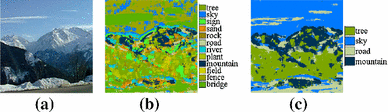Fig. 4

Both the spatial and color terms are computed from the current pixel label estimates. The spatial location term is computed as

\begin{aligned} P_s(\mathbf {x}) = \mathbf {h}_s^{\mathbf {c}_{i}(\mathbf {x})}(\mathbf {x}), \end{aligned}
(5)

where $$\mathbf {h}_s^l(\mathbf {x})$$ is the normalized spatial histogram of word l across all images in the dataset (Fig. 9). This term will assist in places where the appearance and pixel correspondence might not be as reliable.

The color term will assist in refining the labels internally within the image, and is computed as

\begin{aligned} P_{c}^i(\mathbf {x}) = \mathbf {h}_c^{i,\mathbf {c}_i(\mathbf {x})}(I_i(\mathbf {x})) \end{aligned}
(6)

where $$\mathbf {h}_c^{i,l}$$ is the color histogram of word l in image $$I_i$$. We use 3D histograms of 64 bins in each of the color channels to represent $$\mathbf {h}_c^{i,l}$$ instead of the Gaussian mixture models used in Rother et al. (2004) and Shotton et al. (2006).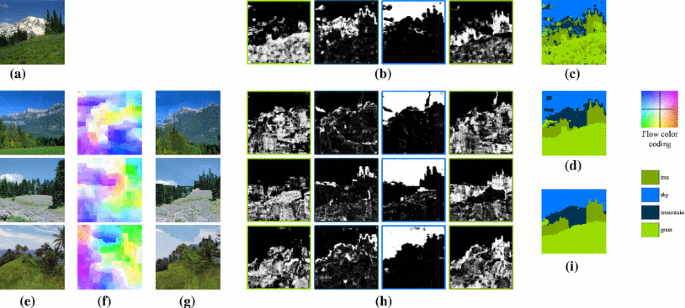Fig. 5

Overall, the parameters of the model are $$\varvec{\Theta }\!=\!\{\mathbf {h}_c^{i,l},\mathbf {h}_s^l,\mathbf {h}_o\}, i=1..N, l=1..L$$.

Regularization The intra-image compatibility between neighboring pixels is defined based on tag co-occurrence and image structures. For image $$I_i$$ and spatial neighbors $$\mathbf {x},\mathbf {y}\in {\mathcal {N}}^{i}_\mathbf {x}$$,

\begin{aligned}&\varPsi ^{i}_{int}(\mathbf {x},\mathbf {y}) = -\lambda _{o} \log \mathbf {h}_{o}\left( \mathbf {c}_i(\mathbf {x}),\mathbf {c}_i(\mathbf {y})\right) \nonumber \\&\quad +\, \delta \left[ \mathbf {c}_i(x) \ne \mathbf {c}_i(\mathbf {y})\right] \lambda _{int}\exp \Big (- \left\| I_i(\mathbf {x}) - I_i(\mathbf {y}) \right\| _2^2\Big ).\nonumber \\ \end{aligned}
(7)

Finally, we define the inter-image compatibility between a pixel $$\mathbf {x}$$ in image $$I_i$$ and its corresponding pixel $$\mathbf {z}=\mathbf {x}+\mathbf {w}_{ij}(\mathbf {x})$$ in image $$I_j$$ as

\begin{aligned}&\varPsi ^{ij}_{\textit{ext}}(\mathbf {x},\mathbf {z}) = \nonumber \\&\quad \delta \left[ \mathbf {c}_i(\mathbf {x}) \ne \mathbf {c}_i(\mathbf {z})\right] \frac{\alpha _j}{\alpha _i} \lambda _{ext} \exp \Big (- \left\| S_i(\mathbf {x}) - S_j(\mathbf {z}) \right\| _1\Big ), \end{aligned}
(8)

where $$\alpha _i,\alpha _j$$ are the image weights as defined in Sect. 4.2.2, and $$S_i$$ are the (dense) SIFT descriptors for image $$I_i$$. Intuitively, better matching between corresponding pixels will result in higher penalty when assigning them different labels, weighted by the relative importance of the neighbor’s label. Notice that SIFT features are used for the inter-image compatibility metric in Eq. 8 whereas RGB intensities are used for the intra-image compatibility in Eq. 7.

### Text-to-Image Correspondence

#### Local Image Descriptors

We selected features used prevalently in object and scene recognition to characterize local image structures and color features. Structures are represented using both SIFT and HOG (Dalal and Triggs 2005) features. We compute dense SIFT descriptors with 3 and 7 cells around a pixel to account for scales. We then compute HOG features and stack together neighboring HOG descriptors within $$2 \times 2$$ patches (Xiao et al. 2010). Color is represented using a $$7 \times 7$$ patch in L*a*b color space centered at each pixel. Stacking all the features yields a 527-dimensional descriptor $$D_i(\mathbf {x})$$ for every pixel $$\mathbf {x}$$ in image $$I_i$$. We use PCA to reduce the descriptor to $$d=50$$ dimensions, capturing approximately $$80\,\%$$ of the features’ variance.

#### Learning Appearance Models

We use a generative model based on Gaussian mixtures to represent the distribution of the above continuous features. More specifically, we model each word in the database vocabulary using a full-covariance Gaussian Mixture Model (GMM) in the 50D descriptor space. Such models have been successfully applied in the past to model object appearance for image segmentation (Delong et al. 2011). Note that our system is not limited to work with this particular model. In fact, we also experimented with a discriminative approach, Randomized Forests (Geurts et al. 2006), previously used for semantic segmentation (Shotton et al. 2008) as an alternative to GMM. We found that GMM produces better results than random forests in our system (see Sect. 4.5).

For pixel $$\mathbf {x}$$ in image $$I_i$$, we define

\begin{aligned} P(D_i(\mathbf {x}); {\varvec{\Theta }})= & {} \sum _{l=1}^L \left( \rho _{l} \sum _{k=1}^M \pi _{l,k} {\mathcal {N}}\left( D_i(\mathbf {x}); {\varvec{\mu }}_{l,k},{\varvec{\Sigma }}_{l,k} \right) \right) \nonumber \\&+\,\rho _{\epsilon } {\mathcal {N}}\left( D_i(\mathbf {x});{\varvec{\mu }}_\epsilon ,{\varvec{\Sigma }}_\epsilon \right) , \end{aligned}
(9)

where $$\rho _l$$ is the weight of model (word) l in generating the feature $$D_i(\mathbf {x}),M$$ is the number of components in each model ($$M=5$$), and $${\varvec{\theta }}_l=\left( \pi _{l,k},{\varvec{\mu }}_{l,k},{\varvec{\Sigma }}_{l,k} \right)$$ is the mixture weight, mean and covariance of component k in model l, respectively. We use a Gaussian outlier model with parameters $${\varvec{\theta }}_{\epsilon } = \left( {\varvec{\mu }}_\epsilon ,{\varvec{\Sigma }}_\epsilon \right)$$ and weight $$\rho _{\epsilon }$$. The intuition for the outlier model is to add an unlabeled word to the vocabulary $${\mathbf {V}}$$. $${{\varvec{\Theta }}}=\left( \{\rho _{l}\}_{l=1:L},\rho _{\epsilon },{\varvec{\theta }}_1,\ldots ,{\varvec{\theta }}_L,{\varvec{\theta }}_\epsilon \right)$$ is a vector containing all parameters of the model.

We optimize for $${{\varvec{\Theta }}}$$ in the maximum likelihood sense using a standard EM algorithm. We initialize the models by partitioning the descriptors into L clusters using k-means and fitting a GMM to each cluster. The outlier model is initialized from randomly selected pixels throughout the database. We also explicitly restrict each pixel to contribute its data to models of words corresponding to its estimated (or given) image tags only. That is, we clamp the posteriors to zero for all $$l\notin {\mathbf {t}}_{i}$$. For labeled images $${\mathbf {I}}_{l}$$, we keep the posteriors fixed according to the given labels (setting zero probability to all other labels). To account for partial annotations, we introduce an additional weight $$\alpha _i$$ for all descriptors of image $$I_i$$, set to $$\alpha _t, \alpha _l$$ or 1 (we use $$\alpha _t=5,\alpha _l=10$$) according to whether image $$I_i$$ was tagged, labeled, or inferred automatically by the algorithm, respectively. More details can be found in the supplementary material. Given the learned model parameters, $${{\varvec{\Theta }}}$$, and an observed descriptor, $$D_i(\mathbf {x})$$, the probability of the pixel belonging to word l is computed by

\begin{aligned} P_a(c_i(\mathbf {x})= & {} l;D_i(\mathbf {x}),{{\varvec{\Theta }}}) \nonumber \\= & {} \frac{\rho _l \sum _{k=1}^M \pi _{l,k} {\mathcal {N}}\left( D_i(\mathbf {x}); {\varvec{\mu }}_{l,k},{\varvec{\Sigma }}_{l,k} \right) }{P(D_i(\mathbf {x}); {{\varvec{\Theta }}})}, \end{aligned}
(10)

where $$P(D_i(\mathbf {x}); {{\varvec{\Theta }}})$$ is defined in Eq. 9.

Figure 5c shows an example pixel classification based on the model learned with this approach, and more results are available on the project web page.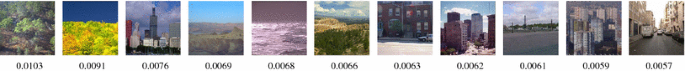Fig. 6

### Optimization

The optimization alternates between estimating the appearance model and propagating pixel labels. The appearance model is initialized from the images and partial annotations in the dataset. Then, we partition the message passing scheme into intra- and inter-image updates, parallelized by distributing the computation of each image to a different core. The belief propagation algorithm starts from spatial message passing (TRW-S) for each image for a few iterations, and then updates the outgoing messages from each image for several iterations. The inference algorithm iterates between message passing and estimating the color histograms in a GrabCut fashion (Rother et al. 2004), and converges in a few iterations. Once the algorithm converges, we compute the MAP labeling that determines both labels and tags for all the images.

### Choosing Images to Annotate

As there is freedom to choose images to be labeled by the user, intuitively, we would want to strategically choose “image hubs” that have many similar images, since such images have many direct neighbors in the image graph to which they can propagate labels efficiently. We use visual pagerank (Jing and Baluja 2008) to find good images to label, again using the Gist descriptor as the image similarity measure. To make sure that images throughout the dataset are considered, we initially cluster the images and use a non-uniform damping factor in the visual rank computation (Eq. 2 in Jing and Baluja 2008), assigning higher weight to the images closest to the cluster centers. Given an annotation budget $$(r_t,r_l)$$, where $$r_t$$ denotes the percentage of images tagged in the dataset, and $$r_l$$ denotes the percentage of the images labeled, we then set $${\mathbf {I}}_l$$ and $${\mathbf {I}}_t$$ as the $$r_l$$ and $$r_t$$ top ranked images, respectively. Figure 6 shows the top image hubs selected automatically with this approach, which nicely span the variety of scenes in the dataset.

### Results

We conducted extensive experiments with the proposed method using several datasets: SUN (Xiao et al. 2010) $$(9556\;256 \times 256\; {\hbox {images}}, 522\;{\hbox {words}})$$, LabelMe Outdoors (LMO, subset of SUN) (Russell et al. 2008) $$(2688\;256 \times 256\;{\hbox {images}}, 33\;{\hbox {words}})$$, the ESP game dataset (Von Ahn and Dabbish 2004) $$(21,846\;{\hbox {images}}, 269\;{\hbox {words}})$$ and IAPR benchmark (Grubinger et al. 2006) (19,805 images, 291 words). Since both LMO and SUN include dense human labeling, we use them to simulate human annotations for both training and evaluation. We use ESP and IAPR data as used by Makadia et al. (2010). They contain user tags but no pixel labeling.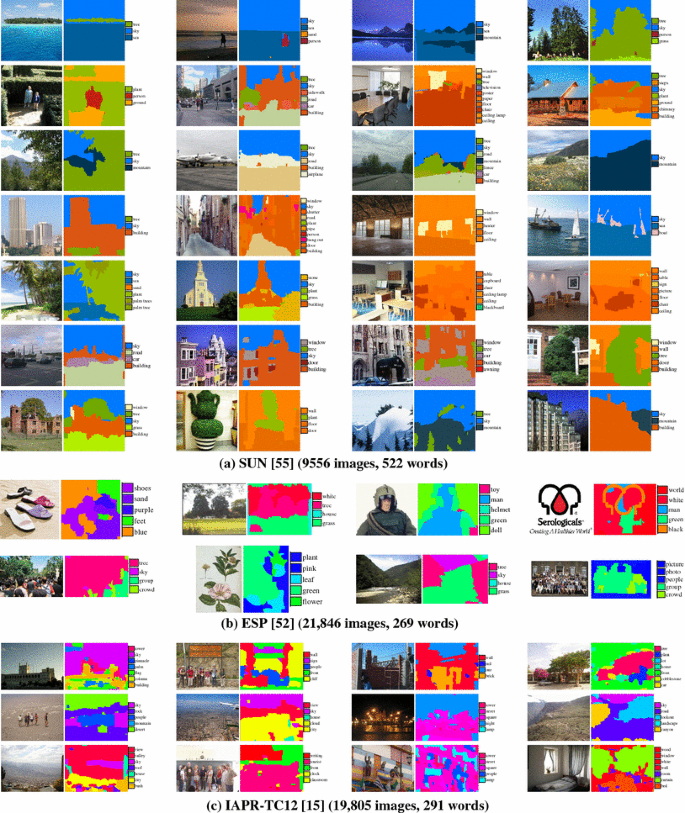Fig. 7

We implemented the system using MATLAB and C++ and ran it on a small cluster of three machines with a total of 36 CPU cores. We tuned the algorithm’s parameters on LMO dataset, and fixed the parameters for the rest of the experiments to the best performing setting: $$\lambda _{s} = 1, \lambda _{c} = 2,\lambda _{o} = 2, \lambda _{int} = 60, \lambda _{ext} =5$$. In practice, 5 iterations are required for the algorithm to converge to a local minimum. The EM algorithm for learning the appearance model (Sect. 4.2.2) typically converge within 15 iterations, and the message passing algorithm (Sect. 4.3) converges in 50 iterations. Using $$K=16$$ neighbors for each image gave the best result (Fig. 10c), and we did not notice significant change in performance for small modifications to $$\epsilon$$ (Sect. 4.1.1), d (Sect. 4.2.1), nor the number of GMM components in the appearance model, M (Eq. 9). For the aforementioned settings, it takes the system 7 h to preprocess the LMO dataset (compute descriptors and the image graph) and 12 h to propagate annotations. The run times on SUN were 15 and 26 h respectively.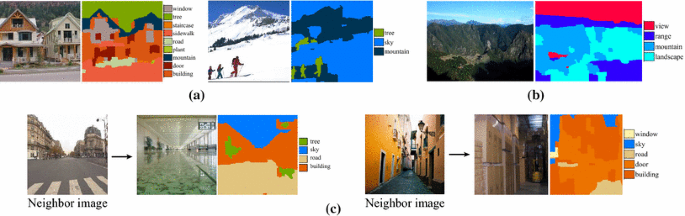Fig. 8

Results on SUN and LMO Figure 7a shows some annotation results on SUN using $$(r_t=0.5,r_l=0.05)$$. All images shown were initially unannotated in the dataset. Our system successfully infers most of the tags in each image, and the labeling corresponds nicely to the image content. In fact, the tags and labels we obtain automatically are often remarkably accurate, considering that no information was initially available on those images. Some of the images contain regions with similar visual signatures, yet are still classified correctly due to good correspondences with other images. More results are available on the project web page.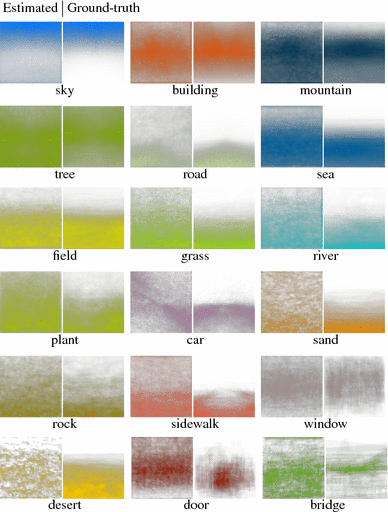Fig. 9

To evaluate the results quantitatively, we compared the inferred labels and tags against human labels. We compute the global pixel recognition rate, r, and the class-average recognition rate $$\bar{r}$$ for images in the subset $$\mathbf {I}\setminus \mathbf {I}_l$$, where the latter is used to counter the bias towards more frequent words. We evaluate tagging performance on the image set $$\mathbf {I}\setminus \mathbf {I}_t$$ in terms of the ratio of correctly inferred tags, P (precision), and the ratio of missing tags that were inferred, R (recall). We also compute the corresponding, unbiased class-average measures $$\bar{P}$$ and $$\bar{R}$$ (Figs. 8, 9).

The global and class-average pixel recognition rates are 63 and $$30\,\%$$ on LMO, and 33 and $$19\,\%$$ on SUN, respectively. In Fig. 10 we show for LMO the confusion matrix and breakdown of the scores into the different words. The diagonal pattern in the confusion matrix indicates that the system recognizes correctly most of the pixels of each word, except for less frequent words such as moon and cow. The vertical patterns (e.g. in the column of building and sky) indicate a tendency to misclassify pixels into those words due to their frequent co-occurrence with other words in that dataset. From the per-class recognition plot (Fig. 10b) it is evident that the system generally performs better on words which are more frequent in the dataset.

Components of the model To evaluate the effect of each component of the objective function on the performance, we repeated the above experiment where we first enabled only the likelihood term (classifying each pixel independently according to its visual signature), and gradually added the other terms. The results are shown in Fig. 10d for varying values of $$r_l$$. Each term clearly assists in improving the result. It is also evident that image correspondences play important role in improving automatic annotation performance.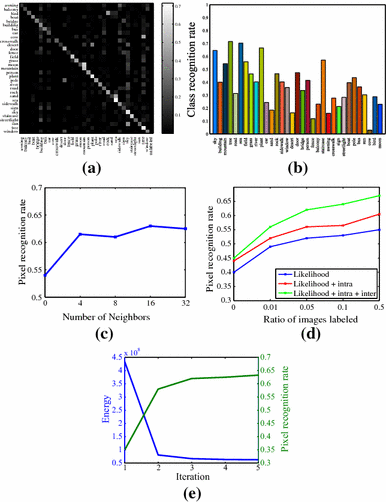Fig. 10

Comparison with state-of-the-art We compared our tagging and labeling results with state-of-the-art in semantic labeling—Semantic Texton Forests (STF) (Shotton et al. 2008) and Label Transfer (Liu et al. 2011a)—and image annotation (Makadia et al. 2010). We used our own implementation of Makadia et al. (2010) and the publicly available implementations of Shotton et al. (2008) and Liu et al. (2011a). We set the parameters of all methods according to the authors’ recommendations, and used the same tagged and labeled images selected automatically by our algorithm as training set for the other methods [only tags are considered by  Makadia et al. (2010)]. The results of this comparison are summarized in Table 1. On both datasets our algorithm shows clear improvement in both labeling and tagging results. On LMO, $$\bar{r}$$ is increased by 5 % compared to STF, and $${\bar{P}}$$ is increased by 8 % over Makadia et al.’s baseline method. STF recall is higher, but comes at the cost of lower precision as more tags are associated on average with each image (Fig. 11). In Liu et al. (2011a), pixel recognition rate of $$74.75\,\%$$ is obtained on LMO at $$92\,\%$$ training/test split with all the training images containing dense pixel labels. However, in our more challenging (and realistic) setup, their pixel recognition rate is $$53\,\%$$ and their tag precision and recall are also lower than ours. Liu et al. (2011a) also report the recognition rate of TextonBoost (Shotton et al. 2006), 52 %, on the same $$92\,\%$$ training/test split they used in their paper, which is significantly lower than the recognition rate we achieve, $$63\,\%$$, while using only a fraction of the densely labeled images. The performance of all methods drops significantly on the more challenging SUN dataset, yet our algorithm still outperforms STF by 10 % in both $${\bar{r}}$$ and $${\bar{P}}$$, and achieves $$3\,\%$$ increase in precision over (Makadia et al. 2010) with similar recall.

We show qualitative comparison with STF in Fig. 11. Our algorithm generally assigns less tags per image compared to STF, for two reasons. First, dense correspondences are used to rule out improbable labels due to ambiguities in local visual appearance. Second, we explicitly bias towards more frequent words and word co-occurrences in Eq. 4, as those were shown to be key factors for transferring annotations (Makadia et al. 2010).

Results on ESP and IAPR We also compared our tagging results with Makadia et al. (2010) on the ESP image set and IAPR benchmark using the same training set and vocabulary they used ($$r_t=0.9,r_l=0$$). Our precision (Table 2) is $$2\,\%$$ better than Makadia et al. (2010) on ESP, and is on par with their method on IAPR. IAPR contains many words that do not relate to particular image regions (e.g. photo, front, range), which do not fit our text-to-image correspondence model. Moreover, many images are tagged with colors (e.g. white), while the image correspondence algorithm we use emphasizes structure. Makadia et al. (2010) assigns stronger contribution to color features, which seems more appropriate for this dataset. Better handling of such abstract keywords, as well as improving the quality of the image correspondences are both interesting directions for future work.

### Training Set Size and Running Time

Training set size. As we are able to efficiently propagate annotations between images in our model, our method requires significantly less labeled data than previous methods (typically $$r_t=0.9$$ in Makadia et al. (2010), $$r_l=0.5$$ in Shotton et al. (2008)). We further investigate the performance of the system w.r.t the training set size on SUN dataset.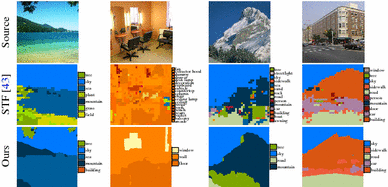Fig. 11

We ran our system with varying values of $$r_t=0.1,0.2,\ldots ,0.9$$ and $$r_l=0.1r_t$$. To characterize the system performance, we use the $$F_1$$ measure, $$F\left( r_t,r_l\right) = \frac{2PR}{P + R}$$ , with P and R the precision and recall as defined above. Following the user study by Vijayanarasimhan and Grauman (2011), fully labeling an image takes 50 s on average, and we further assume that supplying tags for an image takes 20 s. Thus, for example, tagging $$20\,\%$$ and labeling $$2\,\%$$ of the images in SUN requires 13.2 human hours. The result is shown in Fig. 12. The best performance of Makadia et al. (2010), which requires roughly 42 h of human effort, can be achieved with our system with less than 20 human hours. Notice that our performance increases much more rapidly, and that both systems converge, indicating that beyond a certain point adding more annotations does not introduce new useful data to the algorithms.

Running time While it was clearly shown that dense correspondences facilitate annotation propagation, they are also one of the main sources of complexity in our model. For example, for $$10^5$$ images, each 1 mega-pixel on average, and using $$K=16$$, these add $$O(10^{12})$$ edges to the graph. This requires significant computation that may be prohibitive for very large image datasets. To address these issues, we have experimented with using sparser inter-image connections using a simple sampling scheme. We partition each image $$I_i$$ into small non-overlapping patches, and for each patch and image $$j\in N_i$$ we keep a single edge for the pixel with best match in $$I_j$$ according to the estimated correspondence $$\mathbf {w}_{ij}$$. Figure 12 shows the performance and running time for varying sampling rates of the inter-image edges (e.g. 0.06 corresponds to using $$4 \times 4$$ patches for sampling, thus using $$\frac{1}{16}$$ of the edges). This plot clearly shows that the running time decreases much faster than performance. For example, we achieve more than $$30\,\%$$ speedup while sacrificing $$2\,\%$$ accuracy by using only $$\frac{1}{4}$$ of the inter-image edges. Note that intra-image message passing is still performed in full pixel resolution as before, while further speedup can be achieved by running on sparser image grids.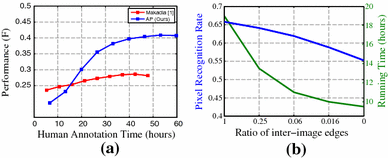Fig. 12

### Limitations

Some failure cases are shown in Fig. 8. Occasionally the algorithm mixes words with similar visual properties (e.g. door and window, tree and plant), or semantic overlap ( e.g. building and balcony). We noticed that street and indoor scenes are generally more challenging for the algorithm. Dense alignment of arbitrary images is a challenging task, and incorrect correspondences can adversely affect the solution. In Fig. 8c we show examples of inaccurate annotation due to incorrect correspondences. More failure cases are available on the project web page.

## Application: Object Discovery and Segmentation

In this section we describe how to use the framework to infer foreground regions among a set of images containing a common object. A particular use case is image datasets returned from Internet search. Such datasets vary considerably in their appearance, and typically include many noise images that do not contain the object of interest (Fig. 2). Our goal is to automatically discover and segment out the common object in the images, with no additional information on the images or the object class.

### Formulation

As we want to label each pixel as either belonging or not belonging to the common object, the dataset vocabulary in this case is comprised of only two labels: $$\mathbf {V}= \{\text {background'', foreground''}\}$$. The pixel labels $$\mathbf {C}= \{\mathbf {c}_1, \ldots , \mathbf {c}_N\}$$ are binary masks, such that $$\mathbf {c}_i(\mathbf {x})=1$$ indicates foreground (the common object), and $$\mathbf {c}_i(\mathbf {x})=0$$ indicates background (not the object) at location $$\mathbf {x}$$ of image $$I_i$$. We also assume no additional information is given on the images or the object class: $$\mathbf {A}= \varnothing$$.

#### Image Graph

We construct the image graph slightly differently than the way it was constructed for annotating images in the previous section. We again use SIFT flow (Liu et al. 2011b) as the core algorithm to compute pixel correspondences, however instead of establishing the correspondence between all pixels in a pair of images as done before, we solve and update the correspondences based on our estimation of the foreground regions. This helps in ignoring background clutter and ultimately improves the correspondence between foreground pixels (Fig. 14), which is a key factor in separating the common object from the background and visual noise.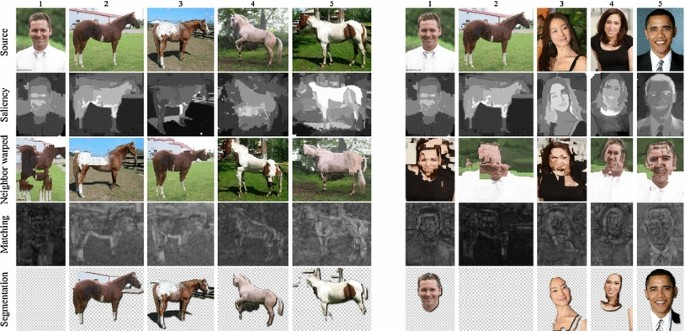Fig. 13

Formally, given (binary masks) $$\mathbf {c}_i, \mathbf {c}_j$$, the SIFT flow objective function becomes

\begin{aligned}&E\left( \mathbf {w}_{ij} ; \mathbf {c}_i,\mathbf {c}_j \right) \nonumber \\&\quad =\sum _{\mathbf {x}\in \varLambda _i} \mathbf {c}_i(\mathbf {x}) \Big ( \mathbf {c}_j(\mathbf {x}+\mathbf {w}_{ij}(\mathbf {x})) \left\| S_i(\mathbf {x}) - S_j(\mathbf {x}+ \mathbf {w}_{ij}(\mathbf {x}))\right\| _1 \nonumber \\&\qquad +\,(1 - \mathbf {c}_j(\mathbf {x}+\mathbf {w}_{ij}(\mathbf {x})) C_0 + \sum _{\mathbf {y}\in \mathcal {N}^i_\mathbf {x}} \alpha \left\| \mathbf {w}_{ij}(\mathbf {x}) - \mathbf {w}_{ij}(\mathbf {y}) \right\| _2 \Big ),\nonumber \\ \end{aligned}
(11)

where $$\mathbf {w}_{ij}$$ is the flow field from image $$I_i$$ to image $$I_j$$, $$S_i$$ are again the dense SIFT descriptors of image $$I_i, \alpha$$ weighs the smoothness term, and $$C_0$$ is a large constant.

The difference between this objective function and the original SIFT flow (Liu et al. 2011b) is that it encourages matching foreground pixels in image $$I_i$$ with foreground pixels in image $$I_j$$. We also use an $$L_2$$-norm for the smoothness term instead of the truncated $$L_1$$-norm in the original formulation (Liu et al. 2011b) to make the flow more rigid in order to surface mismatches between the images. Figure 14(a) shows the contribution of this modification for establishing reliable correspondences between similar images.

We use the Gist descriptor (Oliva and Torralba 2001) to find nearest neighbors, and similarly modify it to account for the foreground estimates by giving lower weight in the descriptor to pixels estimated as background. Figure 14b, c demonstrate that better sorting of the images is achieved when using this weighted Gist descriptor, which in turn improves the set of images with which pixel correspondences are computed.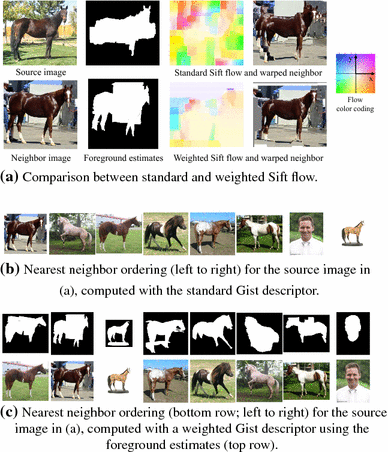Fig. 14

#### Objective Function Terms

Likelihood Our implementation is designed based on the assumption that pixels (features) belonging to the common object should be: (a) salient, i.e. dissimilar to other pixels within their image, and (b) sparse, i.e. similar to pixels (features) in other images with respect to smooth transformations between the images (e.g. with possible changes in color, size and position). The likelihood term is thus defined in terms of the saliency of the pixel, and how well it matches to other pixels in the dataset (Fig. 13):

\begin{aligned} {\varPhi }^i(\mathbf {x}) = \left\{ \begin{array}{ll} {\varPhi }^i_{saliency}(\mathbf {x}) + \lambda _{match} {\varPhi }^i_{match}(\mathbf {x}), &{} \mathbf {c}_i(\mathbf {x}) = 1, \\ \beta , &{} \mathbf {c}_i(\mathbf {x}) = 0, \end{array} \right. \nonumber \\ \end{aligned}
(12)

where $$\beta$$ is a constant parameter for adjusting the likelihood of background pixels. Decreasing $$\beta$$ makes every pixel more likely to belong to the background, thus producing a more conservative estimation of the foreground.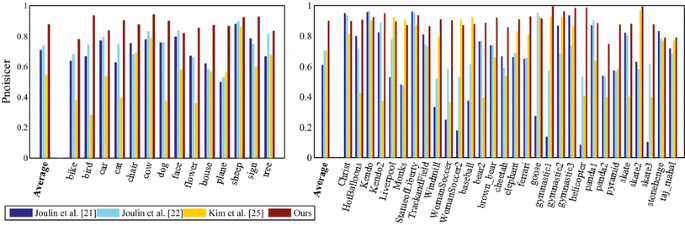Fig. 15

The saliency of a pixel or a region in an image can be defined in numerous ways and extensive research in computer and human vision has been devoted to this topic. In our experiments, we used an off-the-shelf saliency measure—Cheng et al. contrast-based saliency (Cheng et al. 2011)—that produced sufficiently good saliency estimates for our purposes, but our formulation is not limited to a particular saliency measure and others can be used (Figs. 14, 15).

Briefly, Cheng et al. (2011) define the saliency of a pixel based on its color contrast to other pixels in the image (how different it is from the other pixels). Since high contrast to surrounding regions is usually a stronger evidence for saliency of a region than high contrast to far away regions, they weigh the contrast by the spatial distances in the image.

Given a saliency map, $${\widehat{M}}_{i}$$, for each image $$I_i$$, we first compute the dataset-wide normalized saliency, $$M_i$$ (with values in [0, 1]), and define the term

\begin{aligned} {\varPhi }_{saliency}^i\left( \mathbf {x}\right) = -\log M_i(\mathbf {x}). \end{aligned}
(13)

This term will encourage more (resp. less) salient pixels to be labeled foreground (resp. background) later on.

The matching term is defined based on the computed correspondences:

\begin{aligned} \widehat{\varPhi }^i_{match}(\mathbf {x}) = \frac{1}{|N_i|} \sum _{j \in N_i} \left\| S_i(\mathbf {x}) - S_j(\mathbf {x}+ \mathbf {w}_{ij}(\mathbf {x}) \right\| _1, \end{aligned}
(14)

where smaller values indicate higher similarity to the corresponding pixels. Similarly to the saliency, we compute a dataset-wide normalized term $$({\hbox {with values in}} [0,1]), {\varPhi }^i_{match}$$.

Model Parameters We additionally learn the color histograms of the background and foreground of image $$I_i$$, exactly as done in the previous section for propagating annotations (Eq. 6):

\begin{aligned} {\varPhi }^i_\theta (\mathbf {c}_i(\mathbf {x}) = l,\varvec{\Theta }) = -\log \mathbf {h}_c^{i,l}(I_i(\mathbf {x})). \end{aligned}
(15)

Here, the models parameters are comprised of only those color histograms: $$\varvec{\Theta }=\{\mathbf {h}_c^{i,0},\mathbf {h}_c^{i,1}\}, i=1..N$$.

Regularization The regularization terms too are similar to the ones we defined for image annotation (Eqs. 78). Namely, the intra-image compatibility between a pixel, $$\mathbf {x}$$, and its spatial neighbor, $$\mathbf {y}\in \mathcal {N}^i_\mathbf {x}$$, is given by

\begin{aligned} \varPsi _{int}^i(\mathbf {x},\mathbf {y}) = \left[ \mathbf {c}_i(\mathbf {x}) \ne \mathbf {c}_i(\mathbf {y}) \right] \exp \Big (-\Vert I_i(\mathbf {x}) - I_i(\mathbf {y})\Vert _2^2 \Big ), \end{aligned}
(16)

and the inter-image compatibility is defined as

\begin{aligned} \varPsi _{ext}^{ij}(\mathbf {x},\mathbf {z}) = \left[ \mathbf {c}_i(\mathbf {x}) \ne \mathbf {c}_j(\mathbf {z}) \right] \exp \Big (-\Vert S_i(\mathbf {x}) - S_j(\mathbf {z})\Vert _1\Big ),\nonumber \\ \end{aligned}
(17)

where $$\mathbf {z}= \mathbf {x}+ \mathbf {w}_{ij}(\mathbf {x})$$ in the pixel corresponding to $$\mathbf {x}$$ in image $$I_j$$.

### Optimization

The state space in this problem contains only two possible labels for each node: background (0) and foreground (1), which is significantly smaller than the state space for propagating annotations, whose size was in the hundreds. We can therefore optimize equation 1 more efficiently, and also accommodate updating the graph structure within reasonable computational time.

Hence, we alternate between optimizing the correspondences $${\mathbf {W}}$$ (Eq. 11), and the binary masks $${\mathbf {C}}$$ (Eq. 1). Instead of optimizing Eq. 1 jointly over all the dataset images, we use coordinate descent that already produces good results. More specifically, at each step we optimize for a single image by fixing the segmentation masks for the rest of the images. After propagating labels from other images, we optimize each image using a Grabcut-like (Rother et al. 2004) alternation between optimizing equation 1 and estimating the color models $$\{\mathbf {h}_c^{i,0},\mathbf {h}_c^{i,1}\}$$, as before. The algorithm then rebuilds the image graph by recomputing the neighboring images and pixel correspondences based on the current foreground estimates, and the process is repeated for a few iterations until convergence (we typically used 5–10 iterations).

### Results

We conducted extensive experiments to verify our approach, both on standard co-segmentation datasets (Sect. 5.4) and image collections downloaded from the Internet (Sect. 5.5). We tuned the algorithm’s parameters manually on a small subset of the Internet images, and vary $$\beta$$ to control the performance. Unless mentioned otherwise, we used the following parameter settings: $$\lambda _{match}=4, \lambda _{int} = 15, \lambda _{ext} = 1, \lambda _{color}=2, \alpha = 2, K = 16$$. Our implementation of the algorithm is comprised of distributed Matlab and C++ code, which we ran on a small cluster with 36 cores.

We present both qualitative and quantitative results, as well as comparisons with state-of-the-art co-segmentation methods on both types of datasets. Quantitative evaluation is performed against manual foreground-background segmentations that are considered as “ground truth”. We use two performance metrics: precision, P (the ratio of correctly labeled pixels, both foreground and background), and Jaccard similarity, J (the intersection over union of the result and ground truth segmentations). Both measures are commonly used for evaluation in image segmentation research. We show a sample of the results and comparisons in the paper, and refer the interested reader to many more results that we provide in the supplementary material.

### Results on Co-segmentation datasets

We report results for the MSRC dataset (Shotton et al. 2006) (14 object classes; about 30 images per class) and iCoseg dataset (Batra et al. 2010) (30 classes; varying number of images per class), which have been widely used by previous work to evaluate co-segmentation performance. Both datasets include human-given segmentations that are used for the quantitative evaluation.

We ran our method on these datasets both with and without the inter-image components in our objective function (i.e. when using the parameters above, and when setting $$\lambda _{match} = \lambda _{ext} = 0$$, respectively), where the latter effectively reduces the method to segmenting every image independently using its saliency map and spatial regularization (combined in a Grabcut-style iterative optimization). Interestingly, we noticed that using the inter-image terms had negligible effect on the results for these datasets. Moreover, this simple algorithm—an off-the-shelf, low-level saliency measure combined with spatial regularization—which does not use co-segmentation, is sufficient to produce accurate results (and outperforms recent techniques; see below) on the standard co-segmentation datasets!

The reason is twofold: (a) all images in each visual category in those datasets contain the object of interest, and (b) for most of the images the foreground is quite easily separated from the background based on its relative saliency alone. A similar observation was recently made by Vicente et al. (2011), who noticed that their single image classifier outperformed recent co-segmentation methods on these datasets, a finding that is reinforced by our experiments. We thus report the results when the inter-image components are disabled. Representative results from a sample of the classes of each dataset are shown in Fig. 16.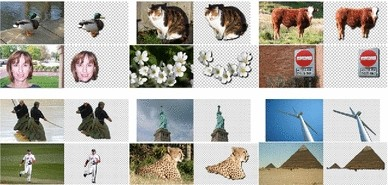Fig. 16

Comparison with Co-segmentation Methods We compared our results with the same three state-of-the-art co-segmentation methods as in Sect. 5.5. The per-class precision is shown in Fig. 15 and the Jaccard similarities are available in the supplemental material on the project web page. Our overall precision ($$87.66\,\%$$ MSRC, $$89.84\,\%$$ iCoseg) shows significant improvement over (Joulin et al. 2012) ($$73.61\,\%$$ MSRC, $$70.21\,\%$$ iCoseg) and (Kim et al. 2011) ($$54.65\,\%$$ MSRC, $$70.41\,\%$$ iCoseg).Fig. 17

Comparison with Object Cosegmentation (Vicente et al. 2011) Vicente et al.’s method (Vicente et al. 2011) is currently considered state-of-the-art on these datasets.Footnote 2 Their code is not publicly available, however they provided us with the segmentation masks for the subsets of MSRC and iCoseg they used in their paper. We performed a separate comparison with their method using only the subset of images they used. Our method outperforms theirs on all classes in MSRC and 9 / 16 of the classes in iCoseg (see supplementary material), and our average precision and Jaccard similarity are slightly better than theirs (Table 3). We note that despite the incremental improvement over their method on these datasets, our results in this case were produced by segmenting each image separately using generic, low-level image cues, while their method segments the images jointly and requires training.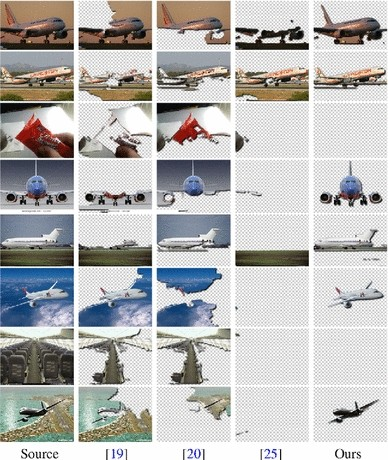Fig. 18

### Results on Internet Datasets

Using the Bing API, we automatically downloaded images for three queries with query expansion through Wikipedia: car (4, 347 images), horse (6, 381 images), and airplane (4, 542 images). With $$K=16$$ nearest neighbors, it took 10 h on average for the algorithm to process each dataset.

Some discovery results are shown in Fig. 17. Overall, our algorithm is able to discover visual objects despite large variation in style, color, texture, pose, scale, position, and viewing-angle. For the objects under a uniform background or with distinct colors, our method is able to output nearly perfect segmentation. Many objects are not very distinctive from the background in terms of color, but they were still successfully discovered due to good correspondences to other images. For car, some car parts are occasionally missing as they may be less salient within their image or not well aligned to other images. Similarly, for horse, the body of horses gets consistently discovered but sometimes legs are missing. More flexible transforms might be needed for establishing correspondences between horses. For airplane, saliency plays a more important role as the uniform skies always match best regardless of the transform. However the algorithm manages to correctly segment out airplanes even when they are less salient, and identifies noise images, such as that of plane cabins and jet engines, as background, since those have an overall worse matching to other images in the dataset.

For qualitative evaluation, we collected partial human labels for each dataset using the LabelMe annotation toolbox (Russell et al. 2008) and a combination of volunteers and Mechanical Turk workers, resulting in 1, 306 car, 879 horse, and 561 airplane images labeled. All labels were manually inspected and refined.

In Table 4 we show the precision and Jaccard similarity of our method on each dataset, with and without using image correspondences. The performance on airplane is slightly better than horse and car as in many of the images the airplane can be easily segmented out from the uniform sky background. Image correspondences helped the most on the car dataset ($$+11\,\%$$ precision, $$+17\,\%$$ Jaccard similarity), probably because in many of the images the cars are not that salient, while they can be matched reliably to similar car images to be segmented correctly.

Comparison with Co-segmentation Methods We compare our results with three previously proposed methods (Joulin et al. 2010, 2012; Kim et al. 2011). For all three methods we used the original implementations by the authors that are publicly available, and verified we are able to reproduce the results reported in their papers when running their code. Since the competing methods do not scale to large datasets, we randomly selected 100 of the images with available ground truth labels from each dataset. We re-ran our method on these smaller datasets for a fair comparison. We also compared to two baselines, one where all the pixels are classified as background (“Baseline 1”), and one where all pixels are classified as foreground (“Baseline 2”). Table 5 summarizes this comparison, showing again that our method produces much better results according to both performance metrics (ours results are not exactly the same as in Table 4 bottom row, since only subsets of the full datasets are used here). The largest gain in precision by our method is on the airplane dataset, which has the highest noise level of these three datasets. Some visual comparisons are shown in Fig. 18 and more are available in the supplementary material.

### Limitations

Some failures of the algorithm are shown in Fig. 17 (last row of each dataset). False positives include a motorcycle and a headlight in the car dataset, and a tree in the horse dataset. This indicates that although matching image structures often leads to object-level correspondence, exceptions occur especially when context is not taken into account.

The algorithm also fails occasionally to discover objects with unique views or background. This is because Gist is a global image descriptor, and unique view and background make it difficult to retrieve similar objects in the dataset.

Finally, our algorithm makes the implicit assumption of non-structured dataset noise. That is, repeating visual patterns are assumed to be part of some “common” object. For example, had a dataset of 100 car images contained 80 images of cars and 20 images of car wheels, then using $$K=16$$ neighbor images by our algorithm may result in intra-group connections, relating images of cars to other images of cars and images of wheels with others alike. In such case the algorithm may not be able to infer that one category is more common than the other, and both cars and wheels would be segmented as foreground. Fortunately, the fixed setting of K we used seems to perform well in practice, however in the general case K needs to be set according to what the user considers as “common”.

## Conclusion

We described an approach for correspondence-driven (a.k.a. example-based) computer vision under sparse training data. We utilize dense image correspondences to construct a large-scale graphical model spanning both labeled and unlabeled images, and solve it to infer pixel labels jointly in all the images by gradually propagating information from labeled images to unlabeled samples.

Our method differs from previous work in three important aspects. First, we use dense image correspondences to explicitly enforce coherent labeling across images, allowing to resolve visual ambiguities due to similar visual patterns. Second, we define an energy function over the entire dataset, and optimize it jointly for all the images. Third, unlike previous approaches which rely on large training sets of densely labeled images, our method can make do with significant less data by efficiently leveraging regularities and structures in the dataset.

We showed how our approach can be applied to two different computer vision problems—image annotation, and automatic visual object discovery and segmentation from the Internet—each of which can be casted as a particular configuration of our general joint inference framework. The machinery we used for solving these problems is very similar, and only requires adapting the objective function terms to the problem at hand (Table 6). We believe our framework can be similarly applied to infer depth, geometry, and edge labels, in large datasets with sparse labeled data.

For image annotation, our experiments show that the proposed system produces reasonable semantic labeling and tagging, and outperforms state-of-the-art methods on several large-scale image dataset while requiring significantly less human annotations. For object discovery, our method is able to naturally handle the visual variation and noise in Internet image collections, and improves upon existing co-segmentation techniques on standard co-segmentation datasets and several challenging Internet datasets.

1. 1.

In our experiments, we did not notice significant difference in the results when computing the nearest neighbor set using pyramid matching (Lazebnik et al. 2006) instead of Gist.

2. 2.

Concurrently to releasing our paper, Kuettel et al. (2012) managed to improve the state-of-the-art precision on the iCoseg dataset ($$91.4\,\%$$).

## References

1. Bagon, S., Brostovski, O., Galun, M., & Irani, M. (2010). Detecting and sketching the common. In IEEE computer society conference on computer vision and pattern recognition (CVPR) (pp. 33–40).

2. Barnes, C., Shechtman, E., Finkelstein, A., & Goldman, D. (2009). Patchmatch: a randomized correspondence algorithm for structural image editing. ACM Transactions on Graphics, 28(3), 24.

3. Batra, D., Kowdle, A., Parikh, D., Luo, J., & Chen, T. (2010). icoseg: Interactive co-segmentation with intelligent scribble guidance. In IEEE computer society conference on computer vision and pattern recognition (CVPR) (pp. 3169–3176).

4. Blei, D. M., Ng, A. Y., & Jordan, M. I. (2003). Latent dirichlet allocation. Journal of Machine Learning Research, 3, 993–1022.

5. Cheng, M. M., Zhang, G. X., Mitra, N. J., Huang, X., & Hu, S. M. (2011). Global contrast based salient region detection. In IEEE computer society conference on computer vision and pattern recognition (CVPR) (pp. 409–416).

6. Collins, M. D., Xu, J., Grady, L., & Singh, V. (2012). Random walks based multi-image segmentation: Quasiconvexity results and gpu-based solutions. In IEEE computer society conference on computer vision and pattern recognition (CVPR) (pp. 1656–1663).

7. Dalal, N., & Triggs, B. (2005). Histograms of oriented gradients for human detection. In IEEE computer society conference on computer vision and pattern recognition (CVPR) (pp. 886–893).

8. Delong, A., Gorelick, L., Schmidt, F. R., Veksler, O., & Boykov, Y. (2011). Interactive segmentation with super-labels. In Energy minimization methods in computer vision and pattern recognition (pp. 147–162).

9. Faktor, A., & Irani, M. (2012). Clustering by composition–unsupervised discovery of image categories. In European conference on computer vision (ECCV) (pp. 474–487).

10. Felzenszwalb, P., McAllester, D., & Ramanan, D. (2008). A discriminatively trained, multiscale, deformable part model. In IEEE computer society conference on computer vision and pattern recognition (CVPR) (pp. 1–8).

11. Feng S, Manmatha R, & Lavrenko, V. (2004). Multiple bernoulli relevance models for image and video annotation. In IEEE computer society conference on computer vision and pattern recognition (CVPR) (pp. II–1002).

12. Fergus, R., Perona, P., & Zisserman, A. (2003). Object class recognition by unsupervised scale-invariant learning. In IEEE computer society conference on computer vision and pattern recognition (CVPR) (Vol. 2, pp. II–264).

13. Freeman, W. T., Pasztor, E. C., & Carmichael, O. T. (2000). Learning low-level vision. International Journal of Computer Vision (IJCV), 40(1), 25–47.

14. Geurts, P., Ernst, D., & Wehenkel, L. (2006). Extremely randomized trees. Machine Learning, 63(1), 3–42.

15. Grubinger, M., Clough, P., Müller, H., & Deselaers, T. (2006). The iapr tc-12 benchmark: A new evaluation resource for visual information systems. In International conference on language resources and evaluation (pp. 13–23).

16. Heath, K., Gelfand, N., Ovsjanikov, M., Aanjaneya, M., & Guibas, L. J. (2010). Image webs: Computing and exploiting connectivity in image collections. In IEEE computer society conference on computer vision and pattern recognition (CVPR) (pp. 3432–3439).

17. Hochbaum, D. S., & Singh, V. (2009). An efficient algorithm for co-segmentation. In IEEE international conference on computer vision (ICCV) (pp. 269–276).

18. Jing, Y., & Baluja, S. (2008). Visualrank: Applying pagerank to large-scale image search. IEEE Transactions on Pattern Analysis and Machine Intelligence (TPAMI), 30(11), 1877–1890.

19. Joulin, A., Bach, F., & Ponce, J. (2010). Discriminative clustering for image co-segmentation. In IEEE computer society conference on computer vision and pattern recognition (CVPR) (pp. 1943–1950).

20. Joulin, A., Bach, F., & Ponce, J. (2012). Multi-class cosegmentation. In IEEE computer society conference on computer vision and pattern recognition (CVPR) (pp. 542–549).

21. Karsch, K., Liu, C., & Kang, S. B. (2012). Depth extraction from video using non-parametric sampling. In European conference on computer vision (ECCV) (pp. 775–788).

22. Kim, G., & Torralba, A. (2009). Unsupervised detection of regions of interest using iterative link analysis. In Advances in neural information processing systems (NIPS) (pp. 961–969).

23. Kim, G., Xing, E. P. (2012). On multiple foreground cosegmentation. In: IEEE computer society conference on computer vision and pattern recognition (CVPR) (pp. 837–844).

24. Kim, G., & Xing, E. P. (2013). Jointly aligning and segmenting multiple web photo streams for the inference of collective photo storylines. In IEEE computer society conference on computer vision and pattern recognition (CVPR) (pp. 620–627).

25. Kim, G., Xing, E. P., Fei-Fei, L., & Kanade, T. (2011). Distributed cosegmentation via submodular optimization on anisotropic diffusion. In IEEE international conference on computer vision (ICCV) (pp. 169–176).

26. Krähenbühl, P., & Koltun, V. (2012). Efficient inference in fully connected crfs with gaussian edge potentials. arXiv preprint arXiv:1210.5644.

27. Kuettel, D., Guillaumin, M., & Ferrari, V. (2012). Segmentation propagation in imagenet. In European conference on computer vision (ECCV) (pp. 459–473).

28. Lazebnik, S., Schmid, C., & Ponce, J. (2006). Beyond bags of features: Spatial pyramid matching for recognizing natural scene categories. In IEEE computer society conference on computer vision and pattern recognition (CVPR) (Vol. 2, pp. 2169–2178).

29. Liang, L., Liu, C., Xu, Y. Q., Guo, B., & Shum, H. Y. (2001). Real-time texture synthesis by patch-based sampling. ACM Transactions on Graphics (ToG), 20(3), 127–150.

30. Liu, C., Yuen, J., & Torralba, A. (2011a). Nonparametric scene parsing via label transfer. IEEE Transactions on Pattern Analysis and Machine Intelligence (TPAMI), 33(12), 2368–2382.

31. Liu, C., Yuen, J., & Torralba, A. (2011b). Sift flow: Dense correspondence across scenes and its applications. IEEE Transactions on Pattern Analysis and Machine Intelligence (TPAMI), 33(5), 978–994.

32. Liu, S., Yan, S., Zhang, T., Xu, C., Liu, J., & Lu, H. (2012). Weakly supervised graph propagation towards collective image parsing. IEEE Transactions on Multimedia, 14(2), 361–373.

33. Makadia, A., Pavlovic, V., & Kumar, S. (2010). Baselines for image annotation. International Journal of Computer Vision (IJCV), 90(1), 88–105.

34. Mukherjee, L., Singh, V., & Dyer, C. R. (2009). Half-integrality based algorithms for cosegmentation of images. In IEEE computer society conference on computer vision and pattern recognition (CVPR) (pp. 2028–2035).

35. Oliva, A., & Torralba, A. (2001). Modeling the shape of the scene: A holistic representation of the spatial envelope. International Journal of Computer Vision (IJCV), 42(3), 145–175.

36. Rother, C., Kolmogorov, V., & Blake, A. (2004). Grabcut: Interactive foreground extraction using iterated graph cuts. ACM Transactions on Graphics (Proc SIGGRAPH), 23(3), 309–314.

37. Rother, C., Minka, T., Blake, A., & Kolmogorov, V. (2006). Cosegmentation of image pairs by histogram matching-incorporating a global constraint into mrfs. In IEEE computer society conference on computer vision and pattern recognition (CVPR) (Vol. 1, pp. 993–1000).

38. Rubinstein, M., Liu, C., & Freeman, W. T. (2012). Annotation propagation in large image databases via dense image correspondence. In European conference on computer vision (ECCV) (pp. 85–99).

39. Rubinstein, M., Joulin, A., Kopf, J., & Liu, C. (2013). Unsupervised joint object discovery and segmentation in internet images. In IEEE computer society conference on computer vision and pattern recognition (CVPR) (pp. 1939–1946).

40. Russell, B. C., Freeman, W. T., Efros, A. A., Sivic, J., & Zisserman, A. (2006). Using multiple segmentations to discover objects and their extent in image collections. In IEEE computer society conference on computer vision and pattern recognition (CVPR) (Vol. 2, pp. 1605–1614).

41. Russell, B. C., Torralba, A., Murphy, K. P., & Freeman, W. T. (2008). Labelme: a database and web-based tool for image annotation. International Journal of Computer Vision (IJCV), 77(1–3), 157–173.

42. Shotton, J., Winn, J., Rother, C., & Criminisi, A. (2006). Textonboost: Joint appearance, shape and context modeling for multi-class object recognition and segmentation. In European conference on computer vision (ECCV) (pp. 1–15).

43. Shotton, J., Johnson, M., & Cipolla, R. (2008). Semantic texton forests for image categorization and segmentation. In IEEE computer society conference on computer vision and pattern recognition (CVPR) (pp. 1–8).

44. Sivic, J., Russell, B. C., Efros, A. A., Zisserman, A., & Freeman, W. T. (2005). Discovering objects and their location in images. In IEEE international conference on computer vision (ICCV) (Vol. 1, pp. 370–377).

45. Snavely, N., Seitz, S. M., & Szeliski, R. (2006). Photo tourism: exploring photo collections in 3D. ACM Transactions on Graphics (Proc SIGGRAPH), 25(3), 835–846.

46. Szeliski, R., Zabih, R., Scharstein, D., Veksler, O., Kolmogorov, V., Agarwala, A., et al. (2008). A comparative study of energy minimization methods for markov random fields with smoothness-based priors. IEEE Transactions on Pattern Analysis and Machine Intelligence (TPAMI), 30(6), 1068–1080.

47. Tappen, M. F., & Liu, C. (2012). A bayesian approach to alignment-based image hallucination. In European conference on computer vision (ECCV) (pp. 236–249).

48. Tighe, J., & Lazebnik, S. (2010). Superparsing: scalable nonparametric image parsing with superpixels. In European conference on computer vision (ECCV) (pp. 352–365).

49. Tompkin, J., Kim, K. I., Kautz, J., & Theobalt, C. (2012). Videoscapes: Exploring sparse, unstructured video collections. ACM Transactions on Graphics, 31(4), 68.

50. Vicente, S., Rother, C., & Kolmogorov, V. (2011). Object cosegmentation. In IEEE computer society conference on computer vision and pattern recognition (CVPR) (pp. 2217–2224).

51. Vijayanarasimhan, S., & Grauman, K. (2011). Cost-sensitive active visual category learning. International Journal of Computer Vision (IJCV), 91(1), 24–44.

52. Von Ahn, L., & Dabbish, L. (2004). Labeling images with a computer game. In ACM conference on human factors in computing systems. Proceedings of SIGCHI (pp. 319–326).

53. Wang, X. J., Zhang, L., Liu, M., Li, Y., & Ma, W. Y. (2010). Arista-image search to annotation on billions of web photos. In IEEE computer society conference on computer vision and pattern recognition (CVPR) (pp. 2987–2994).

54. Winn, J., & Jojic, N. (2005). Locus: Learning object classes with unsupervised segmentation. In IEEE international conference on computer vision (ICCV) (Vol. 1, pp. 756–763).

55. Xiao, J., Hays, J., Ehinger, K. A., Oliva, A., & Torralba, A. (2010). Sun database: Large-scale scene recognition from abbey to zoo. In IEEE computer society conference on computer vision and pattern recognition (CVPR) (pp. 3485–3492).

56. Zhu, S. C., Wu, Y., & Mumford, D. (1998). Filters, random fields and maximum entropy (frame): Towards a unified theory for texture modeling. International Journal of Computer Vision (IJCV), 27(2), 107–126.

57. Zoran, D., & Weiss, Y. (2012). Natural images, gaussian mixtures and dead leaves. In Advances in neural information processing systems (NIPS) (pp. 1736–1744).

Download references

## Acknowledgments

We thank Antonio Torralba for his help in collecting human foreground-background segmentations for our object discovery and segmentation Internet datasets. This work was done while Michael Rubinstein was a Ph.D. student at MIT, supported by the Microsoft Research Ph.D. Fellowship.

## Author information

Authors

### Corresponding author

Correspondence to Michael Rubinstein.

## Additional information

This work was done while Michael Rubinstein was a Ph.D. student at MIT, during his two summer internships at Microsoft Research, and while Ce Liu was a researcher at Microsoft Research.

Communicated by Antonio Torralba and Alexei Efros.

## Rights and permissions

Open Access This article is distributed under the terms of the Creative Commons Attribution 4.0 International License (http://creativecommons.org/licenses/by/4.0/), which permits unrestricted use, distribution, and reproduction in any medium, provided you give appropriate credit to the original author(s) and the source, provide a link to the Creative Commons license, and indicate if changes were made.

Reprints and Permissions